Function Repository Resource:

# ArticulationVertices

Find articulation vertices of a graph

Contributed by: Wolfram Staff (original content by Sriram V. Pemmaraju and Steven S. Skiena)
 ResourceFunction["ArticulationVertices"][g] gives a list of all articulation vertices in graph g.

## Details and Options

Articualtion vertices (or articulation points) are vertices whose removal disconnects the graph.
For directed or mixed graphs, ResourceFunction["ArticulationVertices"][g] is equivalent to ResourceFunction["ArticulationVertices"][UndirectedGraph[g]].

## Examples

### Basic Examples (3)

The only articulation vertex of a star is its center:

 In:=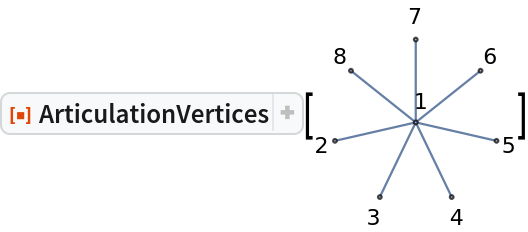Out=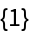All vertices of a path, except the end vertices, are articulation vertices:

 In:=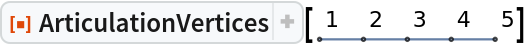Out=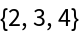Articulation vertices a random graph:

 In:=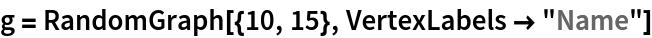Out=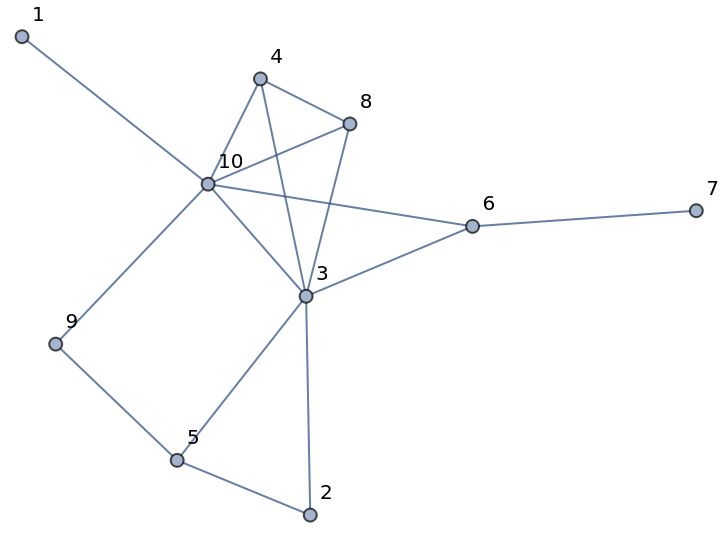In:=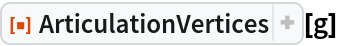Out=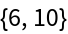In:=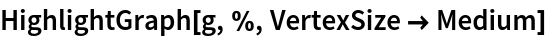Out=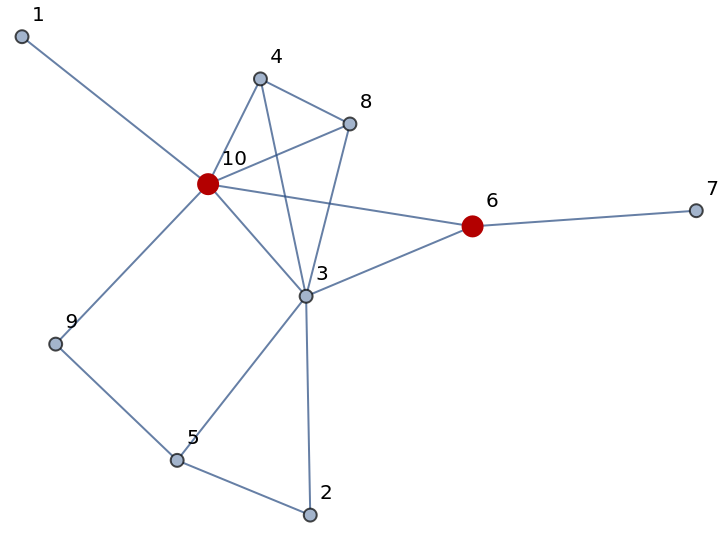### Scope (4)

ArticulationVertices works on undirected graphs:

 In:=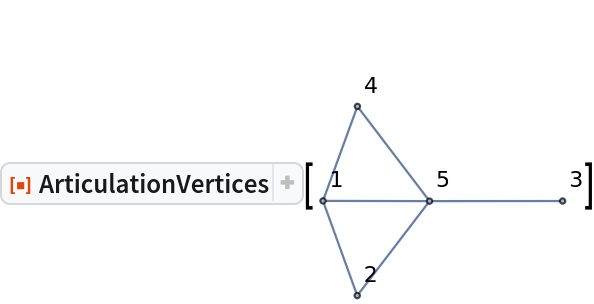Out=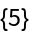Directed graphs:

 In:=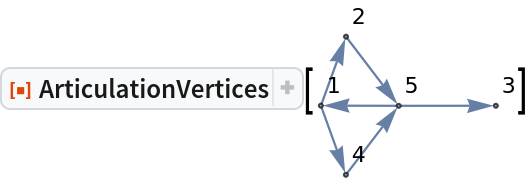Out=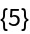Multigraphs:

 In:=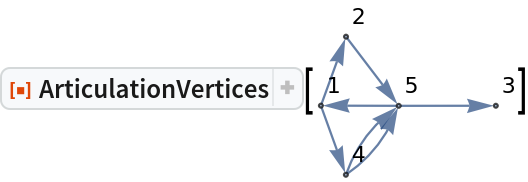Out=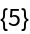Mixed graphs:

 In:=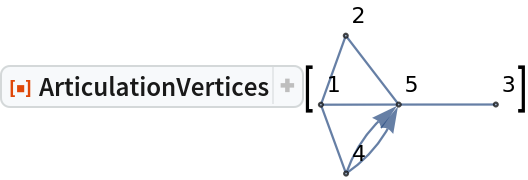Out=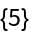### Properties and Relations (7)

Define a graph with multiple articulation vertices:

 In:=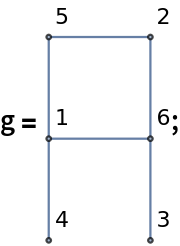In:=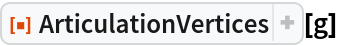Out=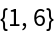Deleting any of the articulation vertices, disconnects the graph:

 In:=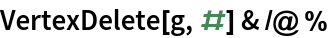Out=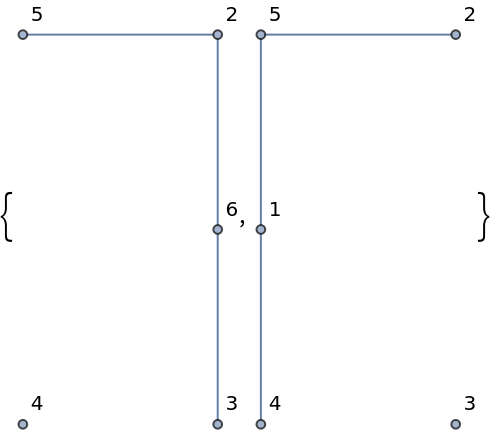In:=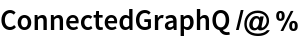Out=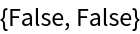FindVertexCut finds a single vertex cut possibly containing multiple vertices:

 In:=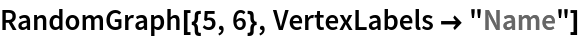In:=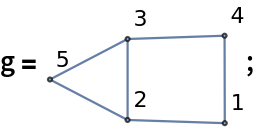In:=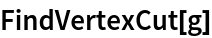Out=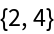For this graph there are no articulation vertices:

 In:=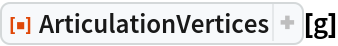Out=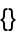For graphs with vertex connectivity 1, the cut vertex returned by FindVertexCut is one of the articulation vertices:

 In:=In:=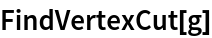Out=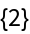In:=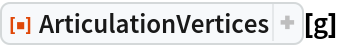Out=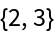A graph with no articulation vertices is biconnected:

 In:=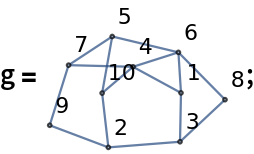In:=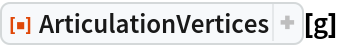Out=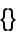In:=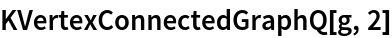Out=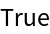A graph with a vertex of degree 1 cannot be biconnected:

 In:=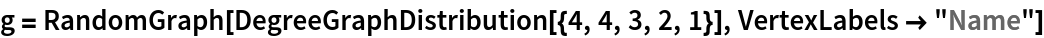Out=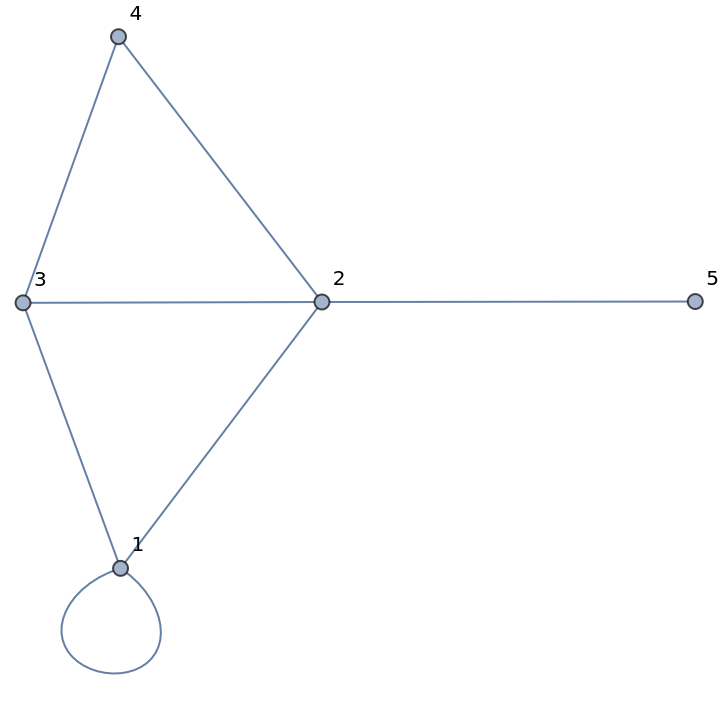In:=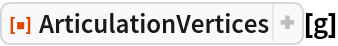Out=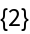An articulation vertex is a member of at least two biconnected components:

 In:=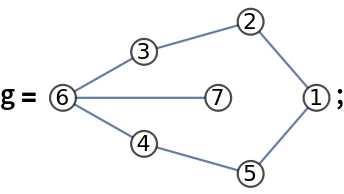In:=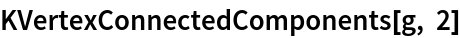Out=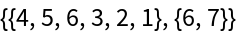In:=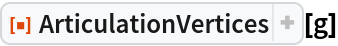Out=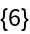GraphData[name,{"Graph","ArticulationVertices"}] gives the articulation vertices of a named graph:

 In:=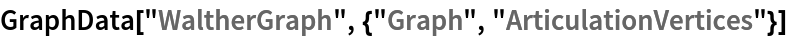Out=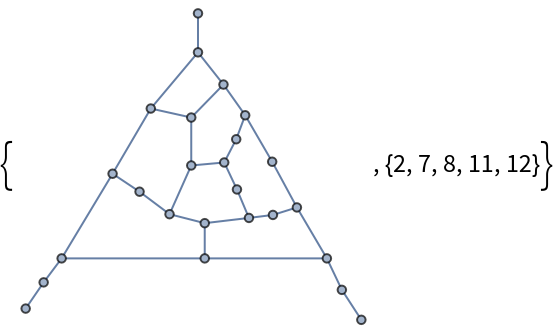In:=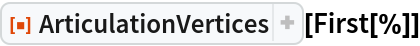Out=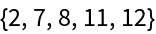## Version History

• 1.0.0 – 13 August 2020